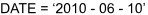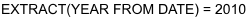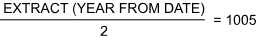Estimating Single-Table Expression Cardinalities | Teradata Vantage - 17.10 - Using Join Index Statistics to Estimate Single-Table Expression Cardinalities - Advanced SQL Engine - Teradata Database

Teradata Vantage™ - SQL Request and Transaction Processing

Product
Release Number
17.10
Release Date
July 2021
Content Type
Programming Reference
User Guide
Publication ID
B035-1142-171K
Language
English (United States)

This topic describes how the Optimizer can use statistics collected on complex expressions specified in the select list of a single-table join index to more accurately estimate the cardinalities of complex expressions specified on base table columns in a query predicate. The use of the term join index anywhere in this topic refers only to single-table join indexes or an equivalent hash index.

You should consider hash indexes to be equivalent to non-sparse single-table join indexes for this topic. Anything written about a non-sparse single-table join index applies equally to an equivalent hash index, substituting the term column list for select list.

Although you cannot collect statistics on complex base table expressions, creating a single-table join index that specifies the same expression in its select list transforms the expression into a simple join index column, and you can then collect statistics on that column. The Optimizer can use those statistics to estimate the single-table cardinality of evaluations of the expression when it is specified in a predicate of a query on the base table.

This capability extends the application of join indexes in query optimization to functions beyond simple query rewrite to include using their statistics for single-table expression cardinality estimation when statistics have not been, or cannot be, collected on complex expressions coded in predicate expressions that refer to their base table columns.

To provide the enhanced single-table expression cardinality estimation capability, the following criteria must be met:
• The join index from which the statistics are collected must specify the relevant complex expression in its select list.
• You must collect statistics on the join index column set that specifies the relevant complex expression.

Definitions of Simple and Complex Expressions

You can collect statistics on a simple join index column created from a complex expression that is specified in its select list. The Optimizer can use statistics collected on the join index column to estimate more accurately the selectivity of complex expressions specified in a query predicate that specifies the matching base table expression.

A simple expression is one with only a simple column reference on the left hand side of the predicate, while a complex expression is one that specifies something other than a simple expression on its left hand side.

For example, the following predicate specifies a simple column reference:The following predicate specifies a complex expression because the term on its left hand side is not a simple column reference:Not all complex expressions can benefit from this optimization. The Optimizer can only use statistics collected on a join index column derived from a complex expression if that expression can be mapped completely to a simple join index expression. The following predicate, for example, cannot be mapped completely to a simple join index expression, so it cannot take advantage of this optimization:This expression cannot be mapped to a simple join index expression on which statistics can be collected.

Matching Predicates and Mapping Expressions

There are several specific cases where join index statistics can provide more accurate cardinality estimates than are otherwise available for base table predicates written using complex date expressions.
• The case where an EXTRACT expression specified in a query predicate can be matched with a join index predicate.
• The case where an EXTRACT DATE expression specified in a query predicate condition can be mapped to an expression specified in the select list of a join index.

The Optimizer uses expression mapping when it detects an identical query expression or a matching query expression subset within a non-matching predicate. When this occurs, the Optimizer maps the predicate to the identical column of the join index, which enables it to use the statistics collected on the join index column to estimate the cardinality of the expression result.

Using Predicate Matching to Estimate Single-Table Cardinalities for Complex Expressions

This topic examines the case where an EXTRACT/DATE expression specified in a query predicate condition can be matched with an expression specified in the select list of a join index. If you have collected statistics on the matching join index column, the Optimizer can then use those statistics to estimate the cardinality of the predicate result.

For example, consider the following join index created on base table t100_a:

CREATE JOIN INDEX ji_a3 AS
SELECT i1, c1
FROM t100_a
WHERE EXTRACT(YEAR FROM d2)>=1969;

You then collect statistics on the default nonunique primary index for ji_a3, ji_a3.i1.

COLLECT STATISTICS ON ji_a3 INDEX (i1);

Join index ji_a3 is designed to support the following queries on base table t100_a:

Query 1

SELECT *
FROM t100_a
WHERE EXTRACT(YEAR FROM d2)>=1969;

Query 2

SELECT *
FROM t100_a
WHERE EXTRACT(YEAR FROM d2)>=1969
AND   i1>2;

Because ji_a3 specifies a predicate (EXTRACT(YEAR FROM d2)>=1969) that is identical to the predicates specified in both queries 1 and 2, and query 2 specifies a predicate condition (i1>2) on a column you have collected statistics on for ji_a3, both queries can use the statistics collected on ji.i1 to enhance the ability of the Optimizer to estimate the cardinalities of their predicate expressions.

The true cardinality for this example is 100 rows.
EXPLAIN SELECT *
FROM t100a
WHERE EXTRACT(YEAR FROM d2)>=1970;
The following shows a portion of the EXPLAIN output:
...
3) We do an all-AMPs RETRIEVE step from df2.t100_a by way of
an all-rows scan with a condition of ("(EXTRACT(YEAR FROM
(df2.t100_a.D2 )))= 1970") into Spool 1 (all_amps), which
is built locally on the AMPs.  The size of Spool 1 is estimated
with high confidence to be 100 rows (49,800 bytes). The estimated
time for this step is 0.03 seconds.

The Optimizer estimates the cardinality of Spool 1 (highlighted in boldface type), which is derived from t_100a, to be 100 rows, an exact match with the true cardinality.

Using Expression Mapping to Estimate Single-Table Cardinalities for Complex Expressions

This topic examines the case where an EXTRACT/DATE expression specified in a query predicate condition can be mapped to an expression specified in the select list of a join index. Mapping converts the EXTRACT expression to an expression written on a DATE column.

When the Optimizer makes a cardinality estimate by mapping from the base table to a join index, the normalization between the base table DATE column that contains the desired year value and the EXTRACT function in the join index definition applies, where join index statistics can provide more accurate cardinality estimates for single-table predicates written using complex expressions specified on the base table than are otherwise available.

The following example demonstrates the case where the query specifies a predicate on DATE column d2, and join index ji_a4 specifies an EXTRACT function on the same DATE column as a simple join index column.

For this case, the Optimizer attempts to convert the predicate DATE condition to its equivalent EXTRACT form before mapping to the join index column. The Optimizer first transforms the query predicate condition d2>=1969-01-01’ to the equivalent expression EXTRACT(YEAR FROM d2)>=‘1969’ and then further transforms that expression to ji.yr>=1969. Because ji.yr>=1969 is a simple expression on a join index column, the Optimizer can use the statistics collected on column ji.yr to estimate the cardinality of the expression result.

Consider the following join index:

CREATE JOIN INDEX ji_a4 AS
SELECT i1, EXTRACT(YEAR FROM d2) AS yr, c1
FROM t100_a
WHERE i1<30;
You then collect the following statistics on the index:
• Column i1 is the default nonunique primary index for ji_a4.
COLLECT STATISTICS ON ji_a4 INDEX(i1);
• The expression alias yr represents the value of the function EXTRACT(YEAR FROM d2).
COLLECT STATISTICS ON ji_a4 COLUMN(yr);

The true cardinality for this example is 30 rows.

The following query specifies a DATE expression in its predicate that can be mapped to the EXTRACT function specified in the select list of join index ji_a4 because d2>=’1969-01-01’ is a matching subset of the ji_a4 select list expression EXTRACT(YEAR FROM d2) AS yr within a non-matching predicate:
EXPLAIN SELECT i1
FROM t100a
WHERE i1<30
AND   d2>='1969-01-01';
The following shows a portion of the EXPLAIN output:
...
3) We do an all-AMPs RETRIEVE step from df2.JI_A4 by way of
an all-rows scan with a condition of ("df2.JI_A4.yr >=
1969") into Spool 1 (all_amps), which is built locally on the AMPs.
The size of Spool 1 is estimated with high confidence to be 30
rows (960 bytes). The estimated time for this step is 0.03
seconds.

The Optimizer estimates the cardinality of Spool 1 (highlighted in boldface type), which is derived from t_100a, to be 30 rows, an exact match with the true cardinality.

Join index ji_a4 is defined to alias the complex expression EXTRACT(YEAR FROM DATE) as yr=2010. This definition enables the Optimizer to map the complex predicate expression EXTRACT(YEAR FROM DATE)=2010 to the simple expression yr=2010, allowing statistics collected on yr to be used to estimate the single-table cardinality of a predicate expression written using base table column references.

But what if you were to code a query predicate expression such as EXTRACT(YEAR FROM DATE)/2 = 1005? The Optimizer can map this expression to the somewhat simpler expression ji_a4.yr/2 = 1005, but this mapping does not enable the use of join index statistics to estimate the single-table cardinality of the expression.

The reason this mapping cannot facilitate more accurate cardinality estimation is that while the mapped expression has a less complicated appearance, it remains a complex expression. Because of the complexity of the expression, the Optimizer cannot use statistics collected on yr to estimate the single-table cardinality of the predicate expression EXTRACT(YEAR FROM DATE)/2=1005 whether it can be mapped to ji_a4.yr/2=1005 or not.

The next example is a slightly more complicated example of expression mapping. This case uses statistics from matching complex expressions specified in the select list of a join index to an expression specified in a query predicate. This example applies to both sparse and non-sparse join indexes, though the join index used for this example is sparse.

Consider the following SELECT request:

SELECT *
FROM perfcurrnew
WHERE BEGIN(vt) <= CURRENT_DATE
AND   END(vt)   >  CURRENT_DATE
AND   END(tt)   IS UNTIL_CHANGED;

Vantage does not support collecting statistics from perfcurrnew on the complex expressions specified in this query predicate that can be used to estimate the cardinality of the result set.

But suppose you create the following join index on perfcurrnew. The select list of this join index specifies expressions that are components of the query predicate written against the base table perfcurrnew in the example SELECT request.

CREATE JOIN INDEX ji, NO FALLBACK, CHECKSUM = DEFAULT AS
SELECT i, j, BEGIN(vt)(AS bvt), END(vt)(AS evt), END(tt)(AS ett)
FROM perfcurrnew
WHERE (evt>DATE)
AND   END(tt) IS UNTIL_CHANGED
PRIMARY INDEX (i);
After creating join index ji, you can collect the following statistics to support the SELECT request on perfcurrnew:
• Column i is the nonunique primary index for ji.
COLLECT STATISTICS ON ji INDEX(i);
• Column vt is defined in base table perfcurrnew with a Period type. The expressions aliased as bvt and evt represent the BEGIN and END bound functions, respectively, of the Period column perfcurrnew.vt, and you can collect statistics on them as they are defined in the join index as ji.bvt and ji.evt.
COLLECT STATISTICS ON ji COLUMN(bvt);
COLLECT STATISTICS ON ji COLUMN(evt);
• Column tt is also defined in perfcurrnew with a Period type, and you can collect statistics on the END bound function of the Period column ji.tt, aliased as ett.
COLLECT STATISTICS ON ji COLUMN(ett);

The true cardinality of the base table perfcurrnew for this example is 691 rows.

The SELECT request in the following example specifies predicates that use the CURRENT_DATE function and the IS UNTIL_CHANGED predicate variable. IS UNTIL_CHANGED represents a “forever” or “until changed” date value for the END Period bound function specified for the Period column perfcurrnew.tt.

Join index ji is defined using a subset of the predicates specified in the SELECT request, so the cardinality estimate for that predicate represents the number of rows selected by the predicates specified on END(vt) and END(tt).

The Optimizer maps the predicate BEGIN(vt)<=CURRENT_DATE-2000 to the expression ji.bvt<=CURRENT_DATE-2000 and uses the statistics collected on ji.bvt.

The combined cardinality estimate made from these statistics represents an evaluation of the cardinality of the result returned by all three of the predicates specified in the original SELECT request.

In this case, the predicates in the query are the same as the predicates specified in the select list of ji, and the cardinality of ji is the same as the cardinality of the result set of the query, so statistics collected on ji are not required to make the cardinality estimate.
EXPLAIN SELECT *
FROM perfcurrnew
WHERE BEGIN(vt) <= CURRENT_DATE - 2000
AND   END(vt)   >  CURRENT_DATE
AND   END(tt)   IS UNTIL_CHANGED;
The following shows a portion of the EXPLAIN output:
...
3) We do an all-AMPs RETRIEVE step from a single partition of
df2.perfcurrnew with a condition of (
"((BEGIN(df2.perfcurrnew.vt ))<= DATE '2004-11-24') AND
(((END(df2.perfcurrnew.tt ))= TIMESTAMP '9999-12-31
23:59:59.999999+00:00') AND ((END(df2.perfcurrnew.vt ))> DATE
'2010-05-17'))") into Spool 1 (all_amps), which is built locally
on the AMPs.  The size of Spool 1 is estimated with high
confidence to be 723 rows (161,952 bytes). The estimated time for
this step is 0.07 seconds.

The estimated cardinality of Spool 1 (highlighted in boldface type), which is derived from perfcurrnew, is 723 rows, which errs by only 32 rows.